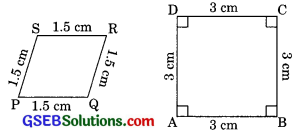# GSEB Solutions Class 10 Maths Chapter 6 Triangle Ex 6.1

Gujarat Board GSEB Solutions Class 10 Maths Chapter 6 Triangle Ex 6.1 Textbook Questions and Answers.

## Gujarat Board Textbook Solutions Class 10 Maths Chapter 6 Triangle Ex 6.1

Question 1.
Fill in the blanks using the correct word given in brackets.

1. All circles are …………. (congruent, similar)
2. All squares are …………. (similar, congruent)
3. All …………. triangles are similar (isosceles, equilateral)
4. Two polygons of the same number of sides are similar. If (a) their corresponding angles are …………. and (b) their corresponding sides are …………. (equal, proportional)

Solution:

1. Similar
2. Similar
3. Equilateral
4. (a) equal (b) proportional.Question 2.
Give two different examples of pair of
(i) Similar figure
(ii) Non-similar figure.
Solution:
(i) (a) Two equilateral triangle are similar.
(b) Two squares are similar.
(ii) (a) Two triangles i.e. one scalene and other isosceles.
(b) Two rectangles i.e. one square and other rectangle.Question 3.
State whether the following quadrilaterals are similar or not.Solution:
Angles of both figure are not equal so they are not similar.